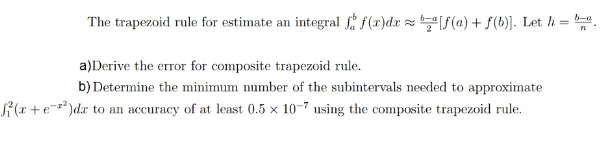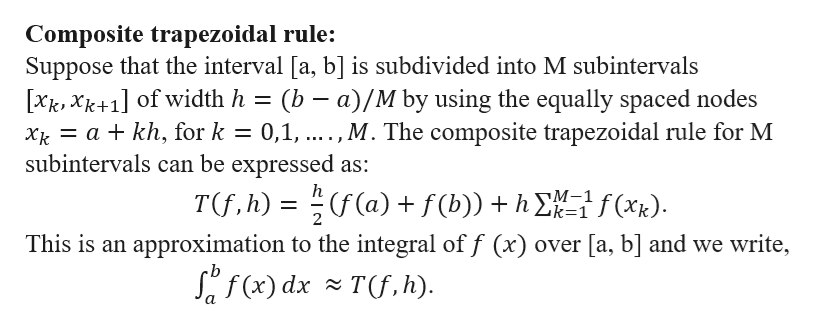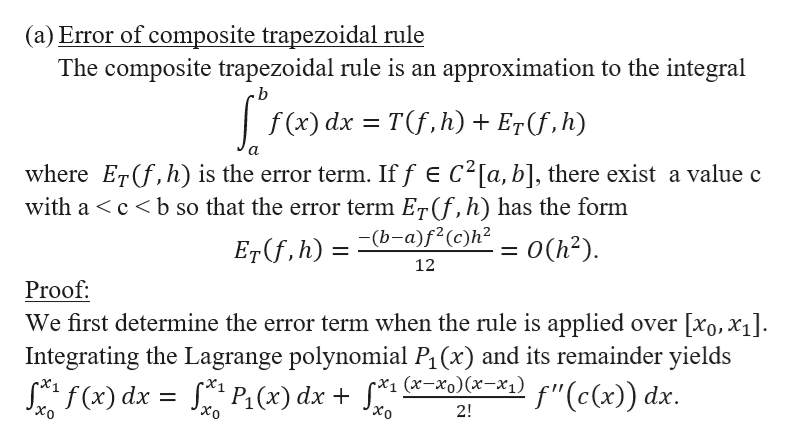# integralf(x)dx z be[f(a) + f(b). Let h = b-aThe trapezoid rule for estimate ana)Derive the error for composite trapezoid ruleb) Determine the minimum number of the subintervals needed to approximate(x+edrto an accuracy of at least 0.5 x 10-7 using the composite trapezoid rule

Question
42 viewshelp_outlineImage Transcriptioncloseintegralf(x)dx z be[f(a) + f(b). Let h = b-a The trapezoid rule for estimate an a)Derive the error for composite trapezoid rule b) Determine the minimum number of the subintervals needed to approximate (x+edr to an accuracy of at least 0.5 x 10-7 using the composite trapezoid rule fullscreen
check_circle

Step 1

Before deriving the error term for composite trapezoidal rule, let us give the statement of composite trapezoidal rule.help_outlineImage TranscriptioncloseComposite trapezoidal rule: Suppose that the interval [a, b] is subdivided into M subintervals [xk,Xk+1] of width h a kh, for k subintervals can be expressed as: (b a)/M by using the equally spaced nodes 0,1,...,M. The composite trapezoidal rule for M h T(f, h) ((a) f(b))h f(xk) M-1 Zk=1 2 This is an approximation to the integral of f (x) over [a, b] and we write, Sf(x) dxT(f, h) fullscreen
Step 2help_outlineImage Transcriptionclose(a) Error of composite trapezoidal rule The composite trapezoidal rule is an approximation to the integral .b f (x) dx Tf,h) + E7(f,h) where ETf,h) is the error term. If f E C2[a, b], there exist a value c with a cb so that the error term Er(f, h) has the form Er(f,h) = (b-a)f<(c)h< _= 0(h2) 12 Proof We first determine the error term when the rule is applied over [xo, x4 Integrating the Lagrange polynomial P1 (x) and its remainder yields Saf(x) dx s P(x) dx + f*1 (*-xo)(x=x°1) f"(c(x)) dx. 2! Xo fullscreen
Step 3

### Want to see the full answer?

See Solution

#### Want to see this answer and more?

Solutions are written by subject experts who are available 24/7. Questions are typically answered within 1 hour.*

See Solution
*Response times may vary by subject and question.
Tagged in

### Math